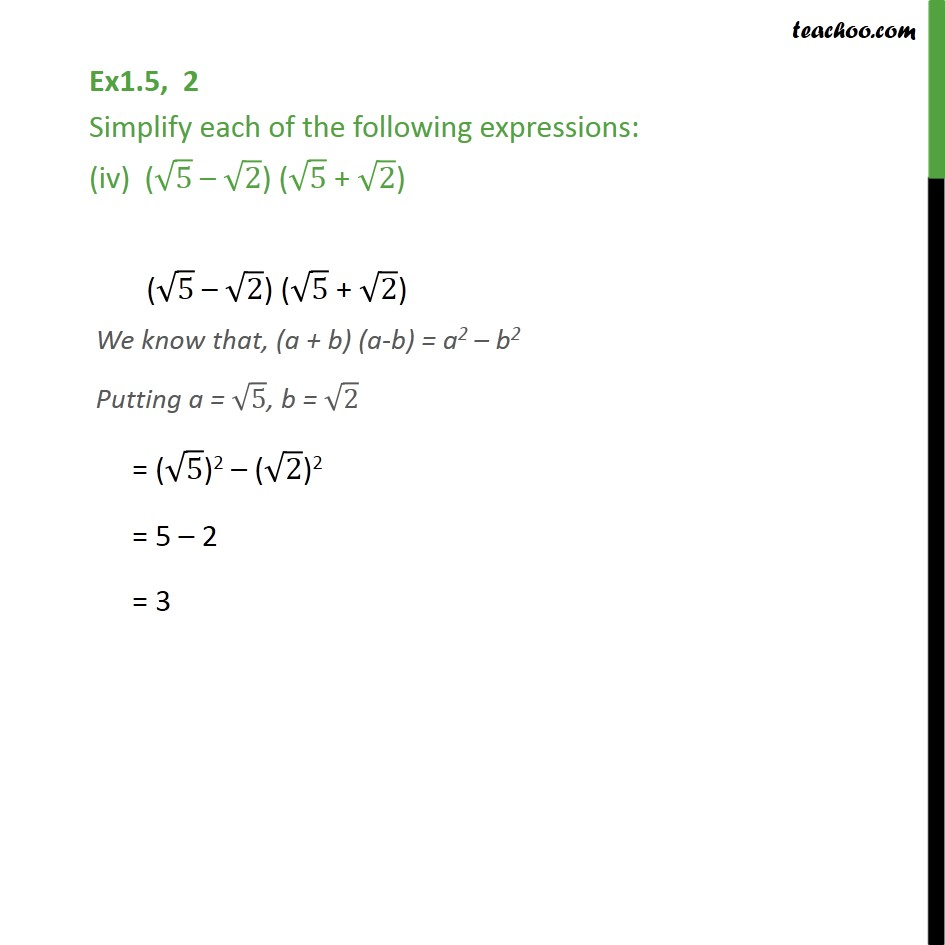Ex 1.4

Chapter 1 Class 9 Number Systems
Serial order wiseLearn in your speed, with individual attention - Teachoo Maths 1-on-1 Class

### Transcript

Ex1.5, 2 Simplify each of the following expressions: (iv) ( 5 2) ( 5 + 2) ( 5 2) ( 5 + 2) We know that, (a + b) (a-b) = a2 b2 Putting a = 5, b = 2 = ( 5)2 ( 2)2 = 5 2 = 3